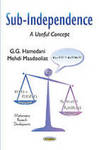## Books by Marquette University Faculty#### Title

Sub-Independence: A Useful Concept

#### Description

The concept of sub-independence is defined in terms of the convolution of the distributions of random variables, providing a stronger sense of dissociation between random variables than that of uncorrelatedness. If statistical tests reject independence but not lack of correlation, a model with sub-independent components can be appropriate to determine the distribution of the sum of the random variables. This monograph presents most of the important classical results in probability and statistics based on the concept of sub-independence. This concept is much weaker than that of independence and yet can replace independence in most limit theorems as well as well-known results in probability and statistics. This monograph, the first of its kind on the concept of sub-independence, should appeal to researchers in applied sciences where the lack of independence of the uncorrelated random variables may be apparent but the distribution of their sum may not be tractable.

#### ISBN

978-1-63463-476-2

2015

#### Publisher

Nova Science Publishers

New York

#### Keywords

Random Variables, Distribution, Probability Theory

#### Disciplines

Mathematics | Probability | Statistical Theory

## Table of Contents

Chapter 1. Introduction

Chapter 2. Some Results on Characterizations Based on the Concept of Sub-Independence

Chapter 3. Central Limit Theorem and Equivalence of Sub-Independence

References

IndexCOinS